Courses

# Boats and Streams - Examples (with Solutions), Logical Reasoning LR Notes | EduRev

## LR : Boats and Streams - Examples (with Solutions), Logical Reasoning LR Notes | EduRev

The document Boats and Streams - Examples (with Solutions), Logical Reasoning LR Notes | EduRev is a part of the LR Course IBPS Clerk Prelims - Study Material, Mock Tests.
All you need of LR at this link: LR

1. A man's speed with the current is 15 km/hr and the speed of the current is 2.5 km/hr. The man's speed against the current is:

A. 8.5 km/hr

B. 10 km/hr.

C. 12.5 km/hr

D. 9 km/hr

Explanation:

Man's speed with the current = 15 km/hr
=> speed of the man + speed of the current = 15 km/hr

speed of the current is 2.5 km/hr
Hence, speed of the man = 15 - 2.5 = 12.5 km/hr

man's speed against the current = speed of the man - speed of the current
= 12.5 - 2.5 = 10 km/hr

2. A motorboat, whose speed in 15 km/hr in still water goes 30 km downstream and comes back in a total of 4 hours 30 minutes. The speed of the stream (in km/hr) is:

A. 10

B. 6

C. 5

D. 4

Explanation:

Speed of the motor boat =15 km/hr
Let speed of the stream =v

Speed downstream =(15+v) km/hr
Speed upstream =(15−v) km/hr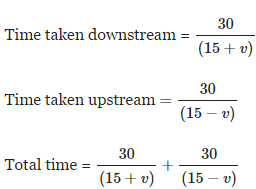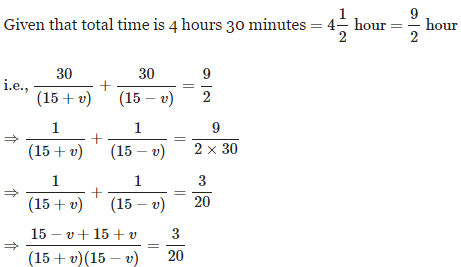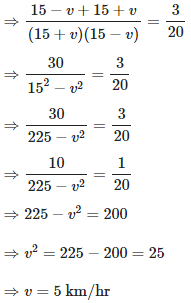3. In one hour, a boat goes 14 km/hr along the stream and 8 km/hr against the stream. The speed of the boat in still water (in km/hr) is:

A. 12 km/hr

B. 11 km/hr

C. 10 km/hr

D. 8 km/hr

Explanation:

Let speed of the boat in still water = a and speed of the stream = b

Then
a + b = 14
a - b = 8

Adding these two equations, we get 2a = 22
=> a = 11

ie, speed of boat in still water = 11 km/hr

4. A man rows to a place 48 km distant and come back in 14 hours. He finds that he can row 4 km with the stream in the same time as 3 km against the stream. The rate of the stream is:

A. 1 km/hr.

B. 2 km/hr.

C. 1.5 km/hr.

D. 2.5 km/hr.

Explanation:

Assume that he moves 4 km downstream in x hours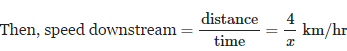Given that he can row 4 km with the stream in the same time as 3 km against the stream

i.e., speed upstream =3/4 of speed downstream

=> speed upstream = 3x km/hr

He rows to a place 48 km distant and comes back in 14 hours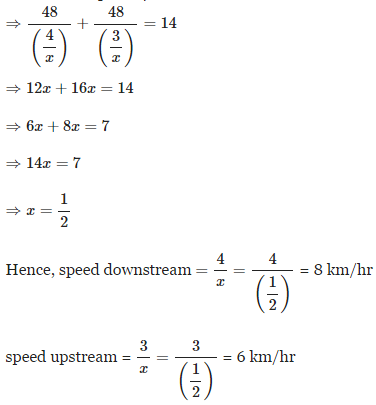Now we can use the below formula to find the rate of the stream

Hence, rate of the stream = 1/2(8−6) = 1km/hr

5. A boatman goes 2 km against the current of the stream in 2 hour and goes 1 km along the current in 20 minutes. How long will it take to go 5 km in stationary water?

A. 2 hr 30 min

B. 2 hr

C. 4 hr

D. 1 hr 15 min

Explanation: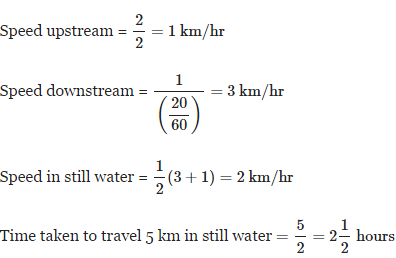= 2 hour 30 minutes

Offer running on EduRev: Apply code STAYHOME200 to get INR 200 off on our premium plan EduRev Infinity!

## IBPS Clerk Prelims - Study Material, Mock Tests

73 videos|43 docs|81 tests

,

,

,

,

,

,

,

,

,

,

,

,

,

,

,

,

,

,

,

,

,

,

,

,

;